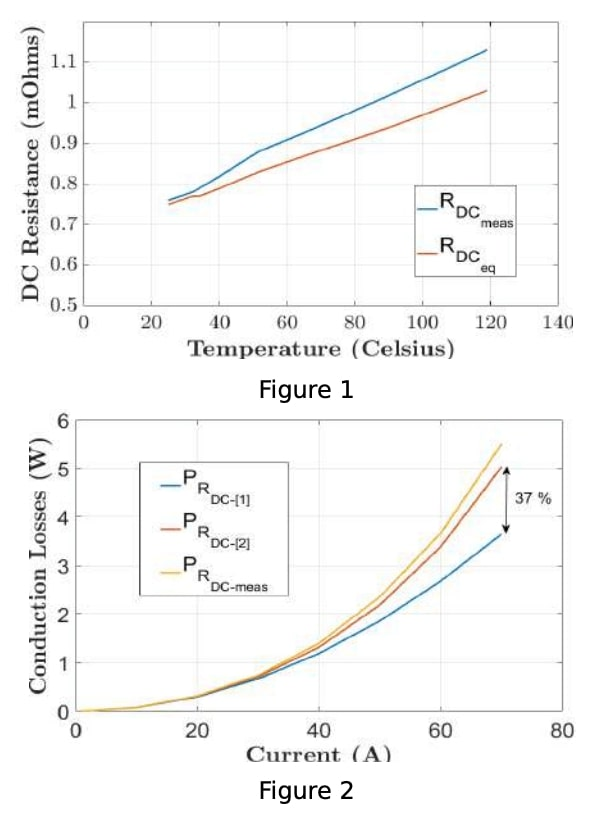# Impact of DC resistance increment with temperature

The well-known conductor's resistance to current flow relationship is given by:

 ???=(? ρ)/?

• l = length of the conductor
• ρ = electrical resistivity of the conductor
• A = Cross-sectional area of the conductor

Such a simple equation has been broadly used to compute conduction losses of magnetic components. However, it is good to remind that electrical resistance is not constant but depends on temperature as given below:

 ?[?]=?[????] (1+α (?−????))

• ?[?] = electric resistance at T
• ?[????] = electric resistance at ????
• α = thermal coefficient of copper resistance

The assumption or simplification of taking RDC value given by  instead of  for power losses analysis could lead to a significant underestimation of the transformer losses and therefore system efficiency.

DC RESISTANCE VS TEMPERATURE TEST

Some test has been done to demonstrate the aforementioned impact of R(T) over the power losses. The test consisted of measuring the RDC vs temperature progression of a round AWG 8 wire while applying different DC current values. Figure 1 shows the theoretical and measured RDCTemperature evolution.There is a maximum error of 8.5 % between theoretical and real measurement mainly due to the accuracy of multimeters and thermal cameras. In any case, the dc resistance increment from 25 ºC to 120 ºC could be up to 37 %. Such an increment is directly reflected in power losses. Figure 2 shows a comparative analysis of the power losses (RI^2) measured during the test with the ones calculated by equations  and .CONCLUSIONS

When designing magnetic components one should be aware of the electrical resistance increment with temperature. Any other simplification could lead to a significant underestimation of the power loss that could lead to malfunction and unexpected results far away from the optimum design.

Do you want the full version?